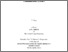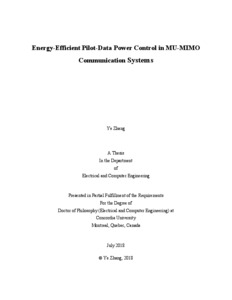Title:

# Energy-Efficient Pilot-Data Power Control in MU-MIMO Communication Systems

Zhang, Ye (2018) Energy-Efficient Pilot-Data Power Control in MU-MIMO Communication Systems. PhD thesis, Concordia University.Preview
Text (application/pdf)
Zhang_PhD_F2018.pdf - Accepted Version
6MB

## Abstract

Multiple-input multiple-output (MIMO) antenna system is considered as a core technology for wireless communication. To reap the benefits of MIMO at a greater scale, massive MIMO with very large antenna arrays deployed at base station (BS) has recently become the forefront in wireless communication research. Till present, the design and analysis of large-scale MIMO systems is a fairly new subject. On the other hand, excessive power usage in MIMO networks is a crucial issue for mobile operators and the explosive growth of wireless services contributes largely to the worldwide carbon footprint. As such, significant efforts have been devoted to improve the spectral efficiency (SE) as well as energy efficiency (EE) of MIMO communication systems over the past decade, resulting in many energy efficient techniques such as power allocation. This thesis investigates novel energy-efficient pilot-data power control strategies which can be used in both conventional MIMO and massive MIMO communication systems. The new pilot-data power control algorithms are developed based ontwo optimization frameworks: one aims to minimize the total transmit power while satisfying per-user signal-interference-plus-noise ratio (SINR) and power constraints; the other aims to maximize the total EE, which is defined as the ratio of the total SE to the transmit power, under individual user power constraints. The proposed novel pilot-data power allocation schemes also take into account the maximum-ratio combining (MRC) and zero-forcing (ZF) detectors in the uplink together with maximum-ratio transmission (MRT) and ZF precoder in the downlink.
Considering that a direct use of such SINR expressions in the power control schemeswould lead to a very difficult optimization problem which is not mathematically tractable, we first investigatethe statistical SINR lower bounds for multi-cell multi-user MIMO (MU-MIMO)communication systemsunder minimum mean square error (MMSE) channel estimation. These lower bounds of the per-user average SINRs are used to replace the true SINRs to simplify the power allocation optimization problems. Such relaxation of the original average SINR yields a simplified problem and leads to a suboptimal solution.
Then, based on the derived average SINR lower bounds, two novel energy efficient pilot-data power control problems are formulatedwithin the first optimization framework,aiming to minimize the total transmit power budget subject to the per-user SINR requirement and power consumption constraint in multi-cell MU-MIMO systems. For the EE-optimal power allocation problems with MRT precoder and MRC detector, it is revealed that such minimization problems can be converted to a standard geometric programming (GP) procedure which can be further converted to a convex optimization problem. For the pilot-data power control scheme with ZF precoder and ZF detector, geometric inequality is used to approximate the original non-convex optimization to GP problem. The very large number of BS station situation is also discussed by assuming infinite antennas at BS. Numerical results validate the tightness of the derived SINR lower bounds and the advantages of the proposed energy efficient power allocation schemes.
Next, two pilot and data power control schemes are developed based on the second power allocation optimization framework to jointly maximize the total EE for both uplink and downlink transmissions in multi-cell MU-MIMO systems under per-user and BS power constraints. The original power control problems are simplified to equivalent convex problems based on the derived SINR lower bounds along with the Dinkelbach's method and the FrankWolfe (FW) iteration. By assuming infinite antennas at BS, the pilot-data power control in massive MIMO case is also discussed. The performance of the proposed pilot-data power allocation schemes based on the two frameworks, namely total transmit power minimization and total EE maximization, are evaluated and compared with the SE maximization scheme.
Furthermore, we investigate the pilot-data power allocation for EE communications in single-cell MU-MIMO systems with circuit power consumption in consideration. The pilot and data power allocation schemes are proposed to minimize the total weighted uplink and downlink transmit power as well as processing circuit power consumption while meeting the per-user SINR and BS power consumption constraints. In our proposed schemes, both fixed and flexible numbers of BS antennas are investigated. For the fixed number of BS antennas case, the non-convex optimization problems are converted to a general GP problem to facilitate the solution. An iterative algorithm is proposed to solve the EE-optimal power control problems in the flexible number of BS antennas casebased on the partial convexity of both the cost function and the constraints. It is shown that the convergence of the proposed iterative algorithm is guaranteed due to the fact that each iteration follows convex optimization.

Divisions: Concordia University > Gina Cody School of Engineering and Computer Science > Computer Science and Software Engineering Thesis (PhD) Zhang, Ye Concordia University Ph. D. Electrical and Computer Engineering 15 July 2018 Zhu, Wei-Ping 984450 YE ZHANG 31 Oct 2018 17:48 02 Apr 2019 15:36
All items in Spectrum are protected by copyright, with all rights reserved. The use of items is governed by Spectrum's terms of access.

Repository Staff Only: item control pageResearch related to the current document (at the CORE website)
Back to top